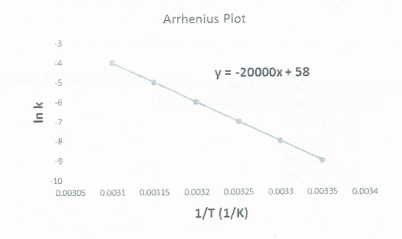# Problem: Using the graph given below, determine the activation energy (E a). R = 8.314 J/mol K a) 166.3 kJ/mol b) 11.6 kJ/mol c) 83.1 kJ/mol d) 130.2 J/mol

###### FREE Expert Solution
90% (109 ratings)
###### Problem Details

Using the graph given below, determine the activation energy (E a). R = 8.314 J/mol K

a) 166.3 kJ/mol

b) 11.6 kJ/mol

c) 83.1 kJ/mol

d) 130.2 J/molWhat scientific concept do you need to know in order to solve this problem?

Our tutors have indicated that to solve this problem you will need to apply the Arrhenius Equation concept. You can view video lessons to learn Arrhenius Equation. Or if you need more Arrhenius Equation practice, you can also practice Arrhenius Equation practice problems.

What is the difficulty of this problem?

Our tutors rated the difficulty ofUsing the graph given below, determine the activation energy...as high difficulty.

How long does this problem take to solve?

Our expert Chemistry tutor, Jules took 2 minutes and 47 seconds to solve this problem. You can follow their steps in the video explanation above.

What professor is this problem relevant for?

Based on our data, we think this problem is relevant for Professor Pelton's class at UMN.